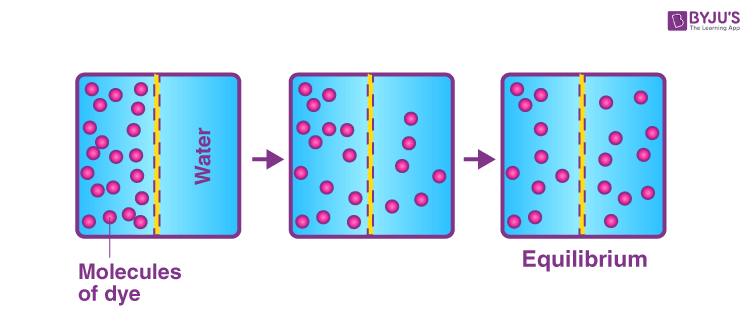Win up to 100% scholarship on Aakash BYJU'S JEE/NEET courses with ABNAT Win up to 100% scholarship on Aakash BYJU'S JEE/NEET courses with ABNAT

# Physical Equilibrium - Types and Examples

Equilibrium indicates the constancy of content and composition (as measured by colour, pressure or temperature) of an item of interest in a system, irrespective of the time period. In the equilibrium state, the rate of the forward reaction is equal to the rate of the backward reaction.

A book on a table, liquid in a closed container, saturated solution, ionic substances in polar solvents and the manufacture of ammonia are some examples of equilibrium.

## What Is Physical Equilibrium?

Physical equilibrium is defined as the equilibrium which develops between different phases or physical properties. In this process, there is no change in chemical composition. It represents the existence of the same substance in two different physical states. The physical equilibrium can be

• Phase equilibrium
• Solute-solid equilibrium
• Gas-liquid equilibriumType of Physical Equilibrium

Let us now discuss the different types of physical equilibrium in detail.

## Types of Physical Equilibrium

### Phase Equilibrium

At 0°C, the number of water molecules becoming ice is equal to the water molecules as the ice melts to form liquid water. The rate of freezing of water is equal to the rate of melting of ice. Thus, there is an equilibrium between solid ice and liquid water.

Ice (s) ⇌ Water (l)

The number of molecules of a liquid becoming vapour will be equal to the number of molecules condensing into liquid in a closed container. The rate of evaporation of liquid water is equal to the rate of condensation of water vapour. The liquid phase is in equilibrium with its own vapour phase.

Water (l) ⇌ Water (g)

### Solute-solid Equilibrium

When a solute in a saturated solution is in contact with an undissolved solute, the number of molecules going (depositing) out of the solution is equal to the number of molecules entering (dissolving) from the solid into the liquid. So the solute in a solution is in equilibrium with the undissolved solid.

Solute (aq) ⇌ Solute (s)

### Gas-liquid Equilibrium

Gases that do not react with liquid may dissolve directly related to the pressure in the liquid. In a closed container, there is an equilibrium between the gas inside the liquid and the gas present above the liquid. In soft drinks, for example, carbon dioxide gas in the liquid is in equilibrium with the gas in the empty space of the container.

Gas (solution) ⇌ Gas (g)

## Examples of Physical Equilibrium

### Examples of Solid-liquid Equilibria

Consider ice and water in a perfectly insulated thermos flask at 0­­­­0C in an open atmosphere. There will be no change in the level of water and quantity of ice, which implies that the rate of transfer of molecules from water to ice is equal to the rate of transfer of molecules from ice to water.

Hence, we can conclude that this system is in a steady state. This can be represented by the following equation:

H2O (s)⇌ H2O (l)

The rate of melting = The rate of freezing

### Examples of Liquid-gas Equilibria

Take distilled water in a closed container and start heating. The water converts into vapour. After a certain time, we will observe that the level of water becomes constant, implying that there is no more conversion of water to vapour and vice-versa.

We can technically say that the rate of evaporation (liquid to vapour) is equal to the rate of condensation (vapour to liquid), thus achieving a steady state. This equation can be represented by the following equation:

H2O (l) ⇌ H2O (g)

The rate of evaporation = The rate of condensation

### Examples of Solid-vapour Equilibria

This kind of equilibrium can exist only in the case of sublimates (solid directly converts to vapour). Consider heating solid iodine in a closed container. Slowly, the vessel is filled with violet-coloured vapour, and the intensity of colour increases with time.

After a certain time, the intensity of colour doesn’t change with time. This implies that a steady state is attained where the rate of sublimation of solid iodine is equal to the rate of deposition of iodine vapour.

I2 (s) ⇌ I2 (Vapour)

The rate of sublimation = The rate of deposition

#### Equilibrium Solved Questions## Frequently Asked Questions – FAQs

Q1

### Define physical equilibrium.

Physical equilibrium is defined as the equilibrium which develops between different phases or physical properties.
Q2

### What are the main types of physical equilibrium?

The main types of physical equilibrium are phase equilibrium, solute-solid equilibrium, and gas-liquid equilibrium.
Q3

### Give one example of equilibrium state.

A liquid in a closed container is an example of equilibrium state.
Test Your Knowledge on Physical Equilibrium